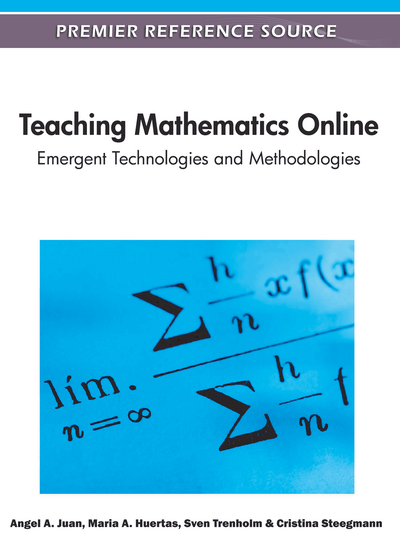# My Equations Are the Same as Yours!: Computer Aided Assessment Using a Gröbner Basis Approach

M Badger (School of Mathematics, University of Birmingham, UK) and C J. Sangwin (Maths, Stats & OR Network, School of Mathematics, University of Birmingham, UK)
DOI: 10.4018/978-1-60960-875-0.ch013
Available
\$37.50
No Current Special Offers

## Abstract

In this chapter we explain how computer aided assessment (CAA) can automatically assess an answer that consists of a system of equations. In particular, we will use a computer algebra system (CAS) and Buchberger’s Algorithm to establish when two systems of equations are the “same.”
Chapter Preview
Top

## Introduction

Our primary concern in this chapter is the assessment of mathematics with computers, and in particular the ability of CAA software to provide automatic feedback in a formative setting to support students. We focus on mathematical methods that allow for the assesment and provision of feedback on questions involving systems of equations. In Section 1 we consider word exercises. These, we argue, are an important component in mathematics education. In Section 2 we discuss computer aided assessment of mathematics and the role of feedback in CAA. Section 3 covers the mathematics underpinning systems of equations. We discuss what it means to solve equations, and when two systems are the same. We examine Euclid's algorithm and Gaussian elimination and then move onto Gröbner basis techniques for manipulating systems of polynomial equations. Section 4 combines the previous topics by explaining how we can automatically assess the modelling component of answers to word exercises using the STACK computer aided assessment system.

## Complete Chapter List

Search this Book:
Reset